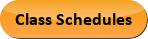Dec 03, 2023
2022-2023 College Catalog
 Select a Catalog 2023-2024 College Catalog 2022-2023 College Catalog [ARCHIVED CATALOG] 2021-2022 College Catalog [ARCHIVED CATALOG] 2020-2021 College Catalog [ARCHIVED CATALOG] 2019-2020 College Catalog [ARCHIVED CATALOG] *** DRAFT 2024-2025 College Catalog DRAFT *** [ARCHIVED CATALOG]
 HELP 2022-2023 College Catalog [ARCHIVED CATALOG] Print-Friendly Page (opens a new window) Add to Portfolio (opens a new window)

# BCT 112 - Construction Mathematics, Communication and Employability

1 Credits, 1 Contact Hours
1 lecture period 0 lab periods

Introduction to basic mathematics concepts and employability in the construction industry. Includes whole numbers, measurements, fractions, decimals, conversion process, metric system, construction geometry, reading, writing, listening and speaking skills, employability in the construction business, critical thinking and computer skills, relationship skills, and workplace issues.

Information: Mathematics assessment test is required before enrolling in this course.Course Learning Outcomes
1. Add, subtract, multiply and divide whole numbers, without a calculator.
2. Use a standard ruler and a metric ruler to measure.
3. Add, subtract, multiply and divide fractions.
4. Add, subtract, multiply and divide decimals, with and without a calculator.
5. Convert decimals to percentages and percentages to decimals, fractions to decimals, and decimals to fractions.
6. Define the metric units of length, weight, volume, and temperature.
7.  Apply basic geometry to measure the basic shapes used in the construction industry.
8. Interpret information and instructions presented in both written and verbal form
9. Describe how to communicate effectively in on-the-job situations using written and verbal skills.
10. Explain entrepreneurship and entering the construction workforce.
11. Solve problems in planning and scheduling for the construction industry.
12. Explain computer systems and common uses of computers in the construction industry.
13. Describe effective relationship skills with teammates and supervisors.
14. Discuss workplace issues involving harassment, stress, and drugs and alcohol.

Outline:
1. Whole Numbers
1. Parts of a whole number
3. Subtracting whole numbers
4. Multiplying simple whole numbers
5. Dividing whole numbers
6. Using the calculator to add, subtract. multiply, and divide whole numbers
2. Measurements
1. Using the standard ruler
2. Architect’s scale
3. Fractions
1. Finding equivalent fractions
2. Reducing fractions to their lowest term
3. Comparing fractions and finding the lowest common denominator
5. Subtracting fractions
6. Multiplying fractions
7. Dividing fractions
4. Decimals
2. Comparing whole numbers with decimals
3. Comparing decimals with decimals
5. Multiplying decimals
6. Dividing with decimals
7. Rounding with decimals
8. Using the calculator to add, subtract, multiply, and divide decimals
5. Conversion Process
1. Converting decimals to percentages and percentages to decimals
2. Converting fractions to decimals
3. Converting decimals to fractions
4. Converting inches to decimal equivalents in feet
6. Metric System
1. Units of weight, length, volume, and temperature
2. Using the metric ruler
3. Converting measurements
7. Construction Geometry
1. Angles
2. Shapes
3. Areas of shapes
4. Volume of shapes
2. Writing on the job
9. Listening and Speaking Skills
1. Active listening on the job
2. Speaking on the job
10. Employability in the Construction Business
1. Entering the construction workforce
2. Entrepreneurship
11. Critical Thinking Skills
1. Barriers to problem solving
2. Solving problems using critical thinking skills
3. Problems with planning and scheduling
12. Computer Skills
1. Computer terms
2. Basic software packages
3. Electronic mail (email)
4. Computers in the construction industry
13. Relationship Skills
1. Self-presentation skills
2. Conflict resolution
3. Giving and receiving criticism
4. Teamwork skills